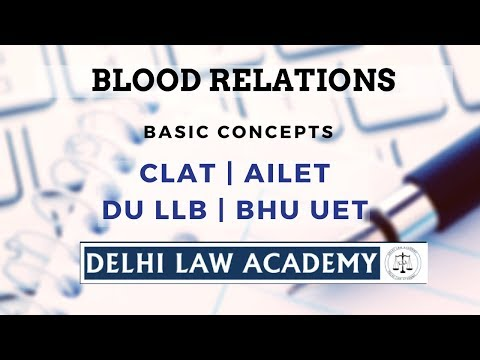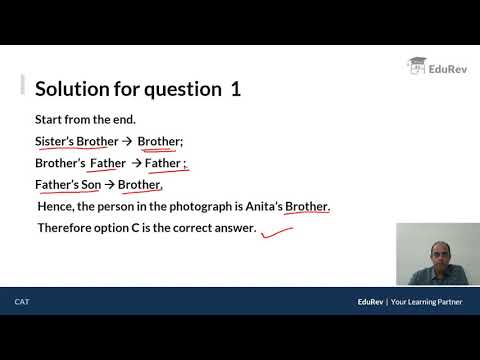Courses

Practice Test: Blood Relations

10 Questions MCQ Test UPSC Prelims Paper 2 CSAT - Quant, Verbal & Decision Making | Practice Test: Blood Relations

Description
Attempt Practice Test: Blood Relations | 10 questions in 10 minutes | Mock test for UPSC preparation | Free important questions MCQ to study UPSC Prelims Paper 2 CSAT - Quant, Verbal & Decision Making for UPSC Exam | Download free PDF with solutions
QUESTION: 1

If A + B means A is the mother of B; A – B means A is the brother B; A % B means A is the father of B and A x B means A is the sister of B, Q. Which of the following shows that P is the maternal uncle of Q?

Solution:

P - M → P is the brother of M
M + N → M is the mother of N
N x Q → N is the sister of Q
Therefore, P is the maternal uncle of Q.

QUESTION: 2

If D is the brother of B, how B is related to C? To answer this question which of the statements is/are necessary? 1.The son of D is the grandson of C. 2.B is the sister of D.

Solution:

Given: D is the brother of B.
From statement 1, we can detect that D is son of C (son of D is the grandson of C).
From statement 2, we can detect that B is 'Female' (sister of D).
Therefore, B is daughter of C.

QUESTION: 3

Introducing a boy, a girl said, “He is the son of the daughter of the father of my uncle.” How is the boy related to the girl?

Solution:

The daughter of the grandfather of the girl will be the sister of her uncle making her the Aunt of the girl. And the son of an aunt would be a cousin brother, hence the answer is Brother.

QUESTION: 4

If A is the brother of B; B is the sister of C; and C is the father of D, how D is related to A?

Solution:

If D is Male, the answer is Nephew.
If D is Female, the answer is Niece.
As the sex of D is not known, hence, the relation between D and A cannot be determined.

QUESTION: 5

If A + B means A is the father of B; A – B means A is the brother B; A % B means A is the wife of B and A x B means A is the mother of B, which of the following shows that M is the maternal grandmother of T?

Solution:

M x N → M is the mother of N

N % S → N is the wife of S

and S + T → is the father of T.

Hence, M is the maternal grandmother of T.

QUESTION: 6

If A + B means A is the brother of B; A x B means A is the son of B; and A % B means B is the daughter of A then which f the following means M is the maternal uncle of N?

Solution:

Because the sex of O is not known.

QUESTION: 7

If A + B means A is the brother of B; A – B means A is the sister of B and A x B means A is the father of B. Which of the following means that C is the son of M?

Solution:

M x N → M is the father of N
N - C → N is the sister of C
and C + F → C is the brother of F.
Hence, M is the father of C or C is the son of M.

QUESTION: 8

Pointing to a photograph. Bajpai said, “He is the son of the only daughter of the father of my brother.” How Bajpai is related to the man in the photograph?

Solution:

The father of Bajpai's brother is Bajpai's father.
Bajpai's father's only daughter is Bajpai's sister.
Bajpai's sister's son is Bajpai's nephew or Bajpai is the maternal uncle of the boy.

QUESTION: 9

Pointing to a photograph of a boy Suresh said, “He is the son of the only son of my mother.” How is Suresh related to that boy?

Solution:

The boy in the photograph is the only son of the son of Suresh's mother i.e., the son of Suresh. Hence, Suresh is the father of boy.

QUESTION: 10

Pointing to a photograph Lata says, “He is the son of the only son of my grandfather.” How is the man in the photograph related to Lata?

Solution:

The man in the photograph is the son of the only son of Lata's grandfather i.e., the man is the son of Lata's father.

Hence, the man is the brother of Lata.Use Code STAYHOME200 and get INR 200 additional OFF Use Coupon Code

How to Prepare for UPSC

Read our guide to prepare for UPSC which is created by Toppers & the best Teachers

Track your progress, build streaks, highlight & save important lessons and more!

Similar ContentRelated tests